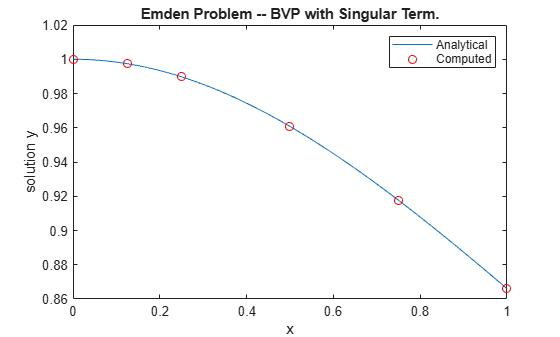Solve BVP with Singular Term

This example shows how to solve Emden's equation, which is a boundary value problem with a singular term that arises in modeling a spherical body of gas.

After reducing the PDE of the model using symmetry, the equation becomes a second-order ODE defined on the interval $\left[0,1\right]$,

${y}^{\prime \prime }+\frac{2}{x}{y}^{\prime }+{y}^{5}=0$.

At $\mathit{x}=0$, the $\left(2/\mathit{x}\right)$ term is singular, but symmetry implies the boundary condition ${\mathit{y}}^{\prime }\left(0\right)=0$. With this boundary condition, the term $\left(2/\mathit{x}\right){\mathit{y}}^{\prime }$ is well defined as $\mathit{x}\to 0$. For the boundary condition $\mathit{y}\left(1\right)=\sqrt{3}/2$, the BVP has an analytical solution that you can compare to a numeric solution calculated in MATLAB®,

$y\left(x\right)={\left[\sqrt{1+\frac{{x}^{2}}{3}}\right]}^{-1}$.

The BVP solver bvp4c can solve singular BVPs that have the form

${y}^{\prime }=S\frac{y}{x}+f\left(x,y\right)$.

The matrix $\mathit{S}$ must be constant and the boundary conditions at $\mathit{x}=0$ must be consistent with the necessary condition $\mathit{S}\cdot \mathit{y}\left(0\right)=0$. Use the 'SingularTerm' option of bvpset to pass the S matrix to the solver.

You can rewrite Emden's equation as a system of first-order equations using ${\mathit{y}}_{1}=\mathit{y}$ and ${\mathit{y}}_{2}={\mathit{y}}^{\prime }$ as

${{\mathit{y}}_{1}}^{\prime }={\mathit{y}}_{2}$,

${{\mathit{y}}_{2}}^{\prime }=-\frac{2}{\mathit{x}}{\mathit{y}}_{2}-{\mathit{y}}_{1}^{5}$.

The boundary conditions become

${\mathit{y}}_{2}\left(0\right)=0$,

${\mathit{y}}_{1}\left(1\right)=\sqrt{3}/2$.

In the required matrix form, the system is

$\left[\begin{array}{c}{{\mathit{y}}_{1}}^{\prime }\text{\hspace{0.17em}}\\ {{\mathit{y}}_{2}}^{\prime }\end{array}\right]=\frac{1}{\mathit{x}}\left[\begin{array}{cc}0& 0\\ 0& -2\end{array}\right]\left[\begin{array}{c}{\mathit{y}}_{1}\\ {\mathit{y}}_{2}\end{array}\right]+\left[\begin{array}{c}{\mathit{y}}_{2}\\ -{\mathit{y}}_{1}^{5}\end{array}\right]$.

In matrix form it is clear that $\mathit{S}=\left[\begin{array}{cc}0& 0\\ 0& -2\end{array}\right]$ and $\mathit{f}\left(\mathit{x},\mathit{y}\right)=\left[\begin{array}{c}{\mathit{y}}_{2}\\ -{\mathit{y}}_{1}^{5}\end{array}\right]$.

To solve this system of equations in MATLAB, you need to code the equations, boundary conditions, and options before calling the boundary value problem solver bvp4c. You either can include the required functions as local functions at the end of a file (as done here), or save them as separate, named files in a directory on the MATLAB path.

Code Equation

Create a function to code the equations for $\mathit{f}\left(\mathit{x},\mathit{y}\right)$. This function should have the signature dydx = emdenode(x,y), where:

• x is the independent variable.

• y is the dependent variable.

• dydx(1) gives the equation for ${{\mathit{y}}_{1}}^{\prime }$, and dydx(2) the equation for ${{\mathit{y}}_{2}}^{\prime }$.

These inputs are automatically passed to the function by the solver, but the variable names determine how you code the equations. In this case:

function dydx = emdenode(x,y)
dydx = [y(2)
-y(1)^5];
end

The term that contains S is passed to the solver using options, so that term is not included in the function.

Code Boundary Conditions

Now, write a function that returns the residual value of the boundary conditions at the boundary points. This function should have the signature res = emdenbc(ya,yb), where:

• ya is the value of the boundary condition at the beginning of the interval.

• yb is the value of the boundary condition at the end of the interval.

For this problem, one of the boundary conditions is for ${\mathit{y}}_{1}$, and the other is for ${\mathit{y}}_{2}$. To calculate the residual values, you need to put the boundary conditions into the form $\mathit{g}\left(\mathit{x},\mathit{y}\right)=0$.

In this form the boundary conditions are

${\mathit{y}}_{2}\left(0\right)=0$,

${\mathit{y}}_{1}\left(1\right)-\sqrt{3}/2=0$.

The corresponding function is then

function res = emdenbc(ya,yb)
res = [ya(2)
yb(1) - sqrt(3)/2];
end

Create Initial Guess

Lastly, create an initial guess of the solution. For this problem, use a constant initial guess that satisfies the boundary conditions, and a simple mesh of five points between 0 and 1. Using many mesh points is unnecessary since the BVP solver adapts these points during the solution process.

${\mathit{y}}_{1}=\sqrt{3}/2$,

${\mathit{y}}_{2}=0$.

guess = [sqrt(3)/2; 0];
xmesh = linspace(0,1,5);
solinit = bvpinit(xmesh, guess);

Solve Equation

Create a matrix for S and pass it to bvpset as the value of the 'SingularTerm' option. Finally, call bvp4c with the ODE function, boundary condition function, initial guess, and option structure.

S = [0 0; 0 -2];
options = bvpset('SingularTerm',S);
sol = bvp4c(@emdenode, @emdenbc, solinit, options);

Plot Solution

Calculate the value of the analytical solution in $\left[0,1\right]$.

x = linspace(0,1);
truy = 1 ./ sqrt(1 + (x.^2)/3);

Plot the analytical solution and the solution calculated by bvp4c for comparison.

plot(x,truy,sol.x,sol.y(1,:),'ro');
title('Emden Problem -- BVP with Singular Term.')
legend('Analytical','Computed');
xlabel('x');
ylabel('solution y');Local Functions

Listed here are the local helper functions that the BVP solver bvp4c calls to calculate the solution. Alternatively, you can save these functions as their own files in a directory on the MATLAB path.

function dydx = emdenode(x,y) % equation being solved
dydx = [y(2)
-y(1)^5];
end
%-------------------------------------------
function res = emdenbc(ya,yb) % boundary conditions
res = [ya(2)
yb(1) - sqrt(3)/2];
end
%-------------------------------------------